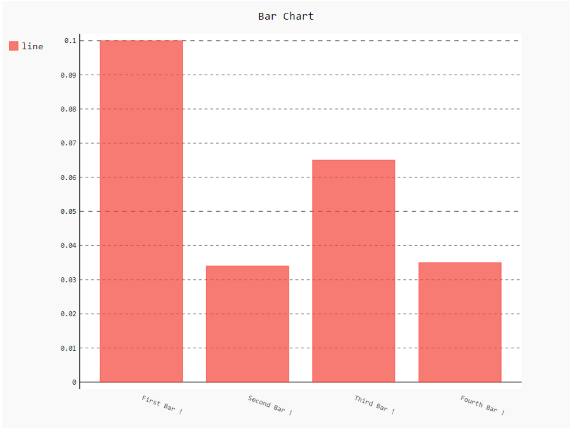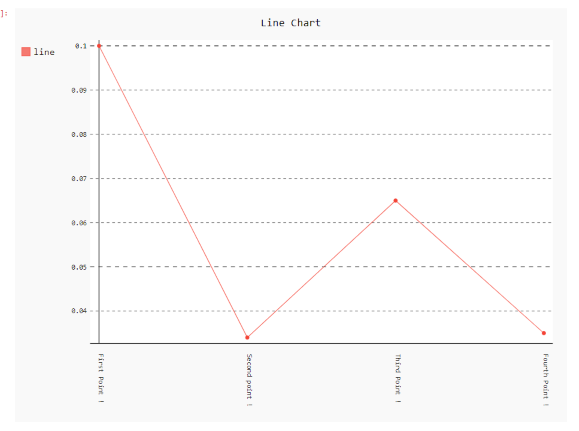# How to rotate x labels using Pygal?

• Last Updated : 24 Jan, 2021

Prerequisites: Pygal

Pygal is a graphics and user interface library for Python that provides functionality commonly required in designing and science applications. While making a plot it is important for us to optimize its label, title, size. In this article, we will see how we can rotate the X-Label value of the plot window in the Pygal module. This can be done by passing the angle of rotation to x_label_rotation parameter.

Attention geek! Strengthen your foundations with the Python Programming Foundation Course and learn the basics.

To begin with, your interview preparations Enhance your Data Structures concepts with the Python DS Course. And to begin with your Machine Learning Journey, join the Machine Learning - Basic Level Course

Here are various ways to change the default plot x_label as per our requirement.

Approach:

• Import required module.
• Create a chart object.
• Pass x_label_rotation value in function.
• Label the graph.
• Display Graph.

Implementation of the concept discussed above is given below:

Example 1:

## Python3

 `# import library``import` `pygal``import` `numpy`` ` `# creating the chart object``chart ``=` `pygal.Bar(x_label_rotation``=``20``)`` ` `# Random data``chart.x_labels ``=` `[``    ``'First Bar !'``,``    ``'Second Bar !'``,``    ``'Third Bar !'``,``    ``'Fourth Bar !'``]``chart.add(``'line'``, [``0.1``, .``034``, .``065``, .``035``])`` ` `# naming the title``chart.title ``=` `'Bar Chart'`` ` `chart.render_to_png(``'img.png'``);`

Output:Example 2:

## Python3

 `# importing pygal``import` `pygal``import` `numpy`` ` `# creating the chart object``chart ``=` `pygal.Line(x_label_rotation``=``90``)`` ` `# Random data``chart.x_labels ``=` `[``    ``'First Point !'``,``    ``'Second point !'``,``    ``'Third Point !'``,``    ``'Fourth Point !'``]``chart.add(``'line'``, [``0.1``, .``034``, .``065``, .``035``])`` ` `# naming the title``chart.title ``=` `'Line Chart'`` ` `chart.render_to_png(``'img.png'``)`

Output:My Personal Notes arrow_drop_up